# Climate Science Glossary

## Term Lookup

Enter a term in the search box to find its definition.

## Settings

Use the controls in the far right panel to increase or decrease the number of terms automatically displayed (or to completely turn that feature off).

# Settings

All IPCC definitions taken from Climate Change 2007: The Physical Science Basis. Working Group I Contribution to the Fourth Assessment Report of the Intergovernmental Panel on Climate Change, Annex I, Glossary, pp. 941-954. Cambridge University Press.HomeArgumentsSoftwareResources CommentsThe Consensus ProjectTranslationsAbout Support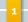Climate's changed before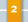It's the sun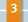It's not bad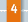There is no consensus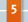It's cooling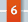Models are unreliable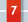Temp record is unreliable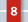Animals and plants can adapt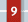It hasn't warmed since 1998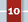Antarctica is gaining ice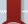View All Arguments...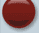## Latest Posts

Archives## A Flanner in the Works for Snow and Ice calculations

#### Posted on 23 January 2011 by MarkR

Endnote and calculations (warning, maths!)

I was always told to show my working so that everyone else could see where I'd gone wrong. So here's some maths for those unfamiliar with climate feedbacks. It's an endnote to my examination of a new paper, those who want to read something interesting should go there.

The change in temperature of the climate in response to a forcing F can be written:

We then define a feedback factor, Y:

Which turns the first equation into:

where Y has been decomposed into a sum of N Yi values, each representing an individual feedback like snow, clouds or water vapour.

We can further decompose each feedback into a product of differentials:

Where ?i is the ith feedback and the differentials explain how it is related to temperature, and how the radiation response is related to it. So far all we've done is use the properties of differentials in an entirely mathematical sense and then made 2 assumptions: that we see an approximately linear response and that the feedback parameter can be split into some linear superposition of individual elements that can be measured.

In the case of snow and ice we need to know its change in albedo for a change in temperature and the change in flux for a change in albedo to calculate Y using the above equation. The change in albedo requires some more information, all of which can be measured by satellite and this is how Flanner et al calculated their feedback parameter.

The first feedback calculated is usually the 'blackbody feedback' - as Earth warms up it radiates more and this has a value of about 3.3 W m-2 K-1. By definition, positive feedbacks are subtracted from this value, whilst negative ones are added (blackbody is positive since it dumps heat from the Earth as temperatures rise). You can use this to do calculations by knowing the initial radiative forcing: a common one is the 3.7 W m-2 you get from doubling CO2. Therefore the 'climate sensitivity' or temperature change from a doubling of CO2, assuming approximate linearity in the range we're looking at so that ?T and ?F can be used is:

where the minus sign comes from the convention of now taking positive feedbacks to be a positive value of Y, and the summation starts at i=2 because I gave the blackbody feedback the index of 1 and it is included as the 3.3 in the denominator.

A climate sensitivity of 3 °C is the classic middle value given by the IPCC. This implies a Y value of about 1.2 using the model results. If Flanner et al's values are correct and are maintained, then global feedback is increased to 0.39 from the current estimate of 0.2, assuming that Southern Hemisphere changes match model predictions. This new value of Y boosts the sensitivity to about 3.5-3.6 °C, which is a 20% increase.

This is, of course, an approximation and I haven't bothered to apply this modified Y to the entire probability distribution function of climate sensitivities, but now you have the tools to DIY!

Further edit

Ken Lambert was unhappy with 'jumping' straight to equilibrium, but wanted to see what happened when you looked at the change moment by moment. So here we go, I'll try the transient climate change case and we will find exactly the same answer as above!

Assume that there is some 'forcing' applied to the climate, dF, and that the Earth changes in response to this. It will warm up or cool down and this will change the rate of heat escape from Earth. e.g. dF causes a warming so the Earth radiates more. I'll call the feedback df.

So the net heat flow dQ is:

However, the feedbacks are a function of temperature, so we can re-write the df using the basic properties of calculus:

And by defining the differential as the letter 'Y' we have our feedback parameter again. By measuring between two finite temperatures, we can take the mean of the path integral of the Y from T1 to T2 as a value of Y that is valid in the equation:

The climate has heat capacity C, and the change in temperature must be the total change in energy in the system (d?Q.dt) divided by the heat capacity, which you can rearrange using the properties of calculus to:

and then substitute this value for ?Q into the original heat flow equation to get a first order differential equation:

This can be solved by integrating factor, and we take t = 0 when ?F = 0. The solution is:

The solution for a general t at constant ?F is:

i.e. as t becomes very large, the exponential tends to 0 so you are left with:

which is the same result as before!

1. I have yet to read the paper, but the press release quoted Flanner as saying that they found an ~+15% increase in TOA forcing. Is that consistent with your calculations? A ~20% sensitivity increase seems huge. Maybe run this by him? BTW, on a related topic I just happened to see this new paper (abstract), which got almost no attention when it came out a couple of weeks ago (I only saw it because of an article in a co-author's hometown newspaper) but sure seems like it deserves some. AFAICT (haven't read the paper) lack of prior data makes it a snapshot rather than a trend analysis, but even so the indicated change (~-25%!) to the land sink is not small potatoes.
2. I agree with Steve Bloom: something is not right here. I think you may have mixed transient response with equilibrium sensitivity. This is quite a worthwhile discussion all the same.
3. These calculations are by definition for the equilibrium climate sensitivity. That's why I said at the bottom 'if the current pattern holds...' So if this relationship between temperature-albedo and albedo-flux holds, then you would expect a boost of 20% to climate sensitivity if your original estimate was 3 C and all other feedbacks retain their properties linearly. This sounds a bit weird, but I'm thinking of doing a post at some point to try and make it clearer. In effect the feedbacks 'reinforce each other' - the extra energy trapped by your change in albedo is 'recycled' multiple times by the enhanced greenhouse effect etc.
4. Thanks Mark. I see that you have a good point. However it is a bit brash to say that certain symbols "by definition" represent an actual physical quantity. Consider this possible physical process: spring now comes earlier snow melts and becomes water wet ground reflects less light than dry ground (I presume here) A century later, spring comes much earlier. Then, by the time each spring when the ground is now wet with melt water, it has dried. Albedo goes back to dry ground albedo. (The wet ground period comes earlier when the sun is a lower angle). Result: equilibrium sensitivity is lower than what you project here.
5. Well, by definition the calculations are for an equilibrium sensitivity (this is true and physical), but what we don't know is whether the value of the feedback parameter we measure now will be the same by the time we reach equilibrium. Which is what your second paragraph is saying! I worded my post saying that if the current measurements are representative of what we ultimately get then this means a big boost to climate sensitivity. But I also tried to make it clear that perhaps it's a case of things just melting more quickly than we expected to begin with and maybe albedo feedback will 'slow down' and head back to model values. All the paper shows is that models underestimate what we expect to see so far, we can probably be confident about that (but ofc we need to see further work for confirmation!) - but more work is needed to have greater confidence that we've underestimated the long term feedback.
6. MarkR: I have an alternative idea. Flanner et al claim that cryosphere albedo feedback is larger than the models have. But those models still track real temperatures (more or less), right? That means that if we include the "correct" feedback, then they (or most of them) would be running too hot, no? Isn't it therefore more likely that something else in the models is off a bit (e.g. aerosol forcing, other feedbacks)?
7. 6 rocco: yes, I think that sounds sensible! Since temperatures roughly follow models, you've pointed out 2 potential consequences: #1 - other feedbacks have been overestimated. I hope so... but other observational studies (Dessler 08/10, Lauer 10, Chung 09) make this seem unlikely; although these are only really preliminary. #2 - the best estimates for forcings are off. If aerosol cooling is stronger than the best estimate (and this is well within the uncertainty) then the original RF is lower and the Y values remain as we have measured them - i.e. higher than models. But there are 2 other potential contributors I can think of: #3 - enhanced heat transport to the deep ocean. #4 - natural noise excluding deep ocean heat transport. Solar activity has declined, as has mean ENSO activity since the '90s. Most of the forcing. After all, the models include approximately a +/- 0.2 C uncertainty and this easily includes a +20% rate of global warming (for the past 30 years that would be +0.1 C or so) so these results don't necessarily mean the models and observations are in disagreement. I reckon you're right, if Flanner's results are good (and they look pretty solid IMO, but we should see if other groups can reproduce them), then it suggests something else is up. Some combination of the above 4 plus probably other stuff could be that something else.
8. MarkR #Original Post Please correct me if I am making a basic error here; but temperature change of a body of mass M and specific heat Sm is proportional to the energy E applied. If we assume that the mass M to be heated is the atmosphere/land/oceans and it has some average specific heat Sa (Joules/kG-degK) and both are roughly constants for this exercise; then M x Sa = K Excuse my lack of maths symbols, but the Temperature change between times 1 and 2 equation looks like this: T2-T1 x K = E2-E1 or Delta T = Delta E / K .......Eqan (1) Delta E = F x delta t; where delta t is the time increment t2-t1 and F is a constant forcing. Therefore; Delta T = F x Delta t / K .......Eqan (2) If F was a variable forcing then F x Delta t would be replaced by the integral of function F wrt t. Perhaps MarkR could take me from Eqan (2) to his end result dT/dF.
9. I don't use equation (2)! If this doesn't work I can write up something with clearer equations. I start by assuming temperature is some function of the heat arriving at the surface in terms of power flux. I work entirely in flux-space, and if you know each element contributing to flux (radiative forcing + feedbacks) then you would have some function to explain it. From the properties of calculus, I get dT = (dT/dF)dF. I used change in flux arriving at the surface and you have used net radiative forcing at the top of the atmosphere - which are very different! When you assume a constant forcing just after (1), you're saying the difference between heat in and heat out is constant all the time. In a world with no feedbacks the world would warm up under a constant forcing and your value of F would actually be an F(t). You made some function of F=F(in)+F(out), which sounds sensible but can actually get very confusing. Whereas I am using some function of F(in). (which =F(out) because I'm considering equilibrium)
10. MarkR: Speaking of underestimated forcings, have you seen this? Could this really be true? Did we have a nuclear mini-winter without anyone noticing?
11. MarkR #9 Energy flux = Power (Forcing) which is the rate of change of energy. Unit is a Watt (Joule/sec) OR a Watt/sq.m. to relate it to a surface aea. Power flux - presumably you mean the rate of change of Power. This would be incremental F/t or differential dF/dt. That must be a Watt/sec or a Joule/sec^2 - right?? "If F was a variable forcing then F x Delta t would be replaced by the integral of function F wrt t." This explains the variable F. You integrate F(t) wrt time 't'. This effectively gives you the area under the F curve - whatever the F function is and this represents the total enegry gained by the mass between times t1 and t2. I can't see these relationships devoid of the time variable 't' which your dF/dT seems to do.
12. Ken Lambert The energy flux is energy over unit surface and over unit time. The energy flux is then not equal to power and its units are J/(m2*s) or W/m2. In your derivation in #8 you omitted the outgoing flux; this is why you get and ever increasing temperature even for a constant forcing, which is unphysical.
13. @ rocco and MarkR: The difference between a climate sensitivity of 3°C or 3.5°C is too low to have such an effect. In other words: Even if you run a model with a sensitivity of 2°C and one with 6°C you reproduce the temperature of the last century quite well with both models! See for example Knutti & Hegerl (2008): "The equilibrium sensitivity of the Earth’s temperature to radiation changes", Nature Geoscience Figure 4: "The observed global warming provides only a weak constraint on climate sensitivity. A climate model of intermediate complexity3, forced with anthropogenic and natural radiative forcing, is used to simulate global temperature with a low climate sensitivity and a high total forcing over the twentieth century (2 °C, 2.5 W m−2 in the year 2000; blue line) and with a high climate sensitivity and low total forcing (6 °C, 1.4 W m−2; red line). Both cases (selected for illustration from a large ensemble) agree similarly well with the observed warming (HadCRUT 3v; black line) over the instrumental period (inset), but show very different long-term warming for SRES scenario A2 (ref. 101)." Thus we have to wait a few years ;-)
14. #11 Ken: my calculations are the effect at equilibrium, they are the result of the integral over time. Your simplistic model is not complete: a more complete model is ΔQ = ΔF - YΔT Where Q is the net flux (which you originally labelled F), F is the radiative forcing and Y is the feedback parameter. For transient calculations, you should use this equation - your original calculation assumed forcing increased at exactly the rate as feedbacks (including heat dumped by the warming Earth's surface). My calculation was for equilibrium, i.e. where ΔQ = 0 and the equation becomes ΔF / Y = ΔT, which is what I put in this post! The time varying nature is included because you take an average Y from the path integral of the feedbacks through temperature space. Also, you don't have to integrate F(t) wrt t because the total energy in the system in the past doesn't necessarily correspond to the temperature today. Analogy: take 2 kettles, boil one and leave the other one cold. Wait a day, then measure their temperatures. Both are the same temperature, even though the integrated energy history of the one that was boiled is higher! Equilibrium is achieved when power in equals power out.
15. #13 MartinS: thanks for the reminder, my response at 7 might be wrong! I stupidly forgot about the transient response: the typical response time τ = C / Y where C is the heat capacity and Y the feedback factor. So response time increases linearly with total warming! An example is an instantaneous forcing at t=0. Assume a typical response time of 10 yr and feedbacks giving 3 C at equilibrium. The approximate response after 10 years is 1.9 C. Assuming that the actual sensitivity is 3.6 C then after 10 years you actually expect 2.0 C. i.e. a 20% boost to equilibrium sensitivity has manifested as a 7% boost after 10 years. So it isn't linear in that sense! This is a simplistic explanation assuming dY/dt = 0 amongst other things, but the IPCC GCMs include these assumptions and it helps explain why we might not be able to detect a higher-than-expected sensitivity in the short term.
16. Rocco @10, Fascinating paper-- just read the abstract. I know that I am not the only one, but I too have often wondered whether the nuclear bombs and tests might have in part been responsible for the slight cooling in global SATs during the mid 20th century.
17. The nuclear winter hypothesis came from fires, not the explosions. Here's an old paper on the topic http://www.atmos.washington.edu/~ackerman/Articles/Turco_Nuclear_Winter_90.pdf
18. #16: "nuclear bombs and tests" I've looked at some of the online records; they actually worked out a formula for height and diameter of cloud vs. yield. Very few of the tests were large enough to put much volume into the stratosphere. '61-'62 was the worst period due to the test ban treaty during '59-'60.
19. MarkR #15 The forcing I labelled 'F' is the net imbalance forcing. That is all the warming forcings minus all the cooling forcings including S-B and WV and Ice albedo feedbacks. My F is the net warming imbalance of 0.9W/sq.m currently (09)estimated in the Trenberth paper (Aug09). Riccardo #12 == see above. Energy/time = Power which has the unit Watt (Joule/sec). The unit of area simply divides it into a rate per sq.m. "In your derivation in #8 you omitted the outgoing flux; this is why you get and ever increasing temperature even for a constant forcing, which is unphysical" My forcing F is the *net* forcing as described above. It will not remain constant as the various component forcings change. The main cooling forcing S-B will rise with T^4, so F will be a complex function which steadily approaches zero as temperature equilibrium is reached. ie: "You integrate F(t) wrt time 't'. This effectively gives you the area under the F curve - whatever the F function is and this represents the total energy gained by the mass between times t1 and t2." In reality at time t2, F approaches zero and the total energy absorbed by the Earth system will be equal to the area under the F curve representing (1) equilibrium Temperature rise x specific heat of the masses heated; plus (2) phase change heat of ice melted; plus (3) latent heat of extra water evaporated.
20. Ken Lambert your definition of forcing as including, to make it short, everything is quite uncommon; MarkR definition, which is kind of standard, is different. Anyways, given your definition, your eq. 2 in #8 is wrong since it applies only for constant F which is the case only in steady state. Beware, it does not need to "steadily approaches zero as temperature equilibrium is reached". If for example if you have a linearly increasing (in time) forcing (using the standard definition), your F will aproach a constant value different from zero.
21. Riccardo #20 Eqan (2) is not wrong. Read this again.... ""Delta E = F x delta t; where delta t is the time increment t2-t1 and F is a constant forcing. Therefore; Delta T = F x Delta t / K .......Eqan (2) If F was a variable forcing then F x Delta t would be replaced by the integral of function F wrt t."" You can call my 'F' - 'Q' if you like - it does not alter the relationships. I have already covered the case where F is a variable function over time. If I had an integral symbol on my keyboard I could substitute "Fconstant x Delta t" with "integral of function F wrt t". I don't think that you understand that F is the forcing imbalance. It is the net of all the forcings operating on the climate system. In a steady state F will be zero by definition. In a warming state F will be positive and in a cooling state F will be negative.
22. Ken Lambert read this again ... "your eq. 2 in #8 is wrong since it applies only for constant F which is the case only in steady state." I think you should put more effort in the definition of the quantities you use. Compare this: "It is the net of all the forcings operating on the climate system." with this "That is all the warming forcings minus all the cooling forcings including S-B and WV and Ice albedo feedbacks." Please clarify what you mean by forcing and what by feedback, I maybe got confused by your ambiguity in the definitions.
23. #21: Ken, I think I got confused when you used different conventions to 'standard', and you switched definitions as well! I've added a note to the main body that derives the original ΔT = ΔF/Y equilibrium equation by considering the heat flow through time like you wanted to do. This includes both forcings and feedbacks and considers the net heat flow at any point in what I'm pretty sure is the correct manner. As you see, it's messier than just considering the fluxes at equilibrium and since both approaches are valid, they give the same answer! Hopefully this will be interesting. :)
24. MarkR #23 and Riccardo #22 So how large does time 't' become in order for equilibrium to be reached given the non-linear multi-factored components of Delta F (presumably equal to my F.imbalance), and your assumed value (or function) for Y? Given that my current F.imbalance = F.CO2GHG + F.otherGHG - F.cloud albedo - F.direct albedo + F.solar - F.radiative feedbackSB + F.WVIA feedback..........= 0.9W/sq.m Ref; Trenberth Fig 4 "Tracking the Earth's Global Energy" Aug09. (WVIA - water vapour + ice albedo feedbacks). To know where the above sum will go into the future; one would need a function for each - something I have never seen for F.cloud albedo or F.WVIA feedback).
25. ΔF is not your imbalance, ΔQ is. ΔF is radiative forcing; heat flow that doesn't react to the climate state on the timescales we're considering. Changes in CO2, volcanism and solar activity are examples. YΔT is the feedback sum that responds to the state of the climate. We have estimates of sensitivity (and therefore net Y in the past) from palaeoclimate. We have direct measurements of Y from the past few decades and climate models implicitly calculate it So far climate models have generally been in agreement, or underestimated observational values.
26. Ken Lambert the response time τ in a simple zero-dimensional model is given by τ=C/λ whre C is the heat capacity and λ-1 is the climate sensitivity. Howver, this model is a bit crude. Indeed, there are several response times relative to the various parts of the climate system. As a grossly aproximated single "effective" response time you can take something like 40-50 years. To have the time dependence of forcings and feedbacks you need to run a climate model, better if many runs of many models; something I have never seen either.
27. MarkR #25 So Delta F should equate to my F.CO2GHG + F.otherGHG + F.solar (which are all the supposed independent of temperature) Y x DeltaT should equate to the climate and temperature responses: F.WVIA feedback - F.radiative feedbackSB - F.cloud albedo - F.direct albedo. ?? With your Delta Q equal to the difference between the two above terms. Is that right?
28. That looks right to me! Be careful with sign conventions: to calculate total Y 'normal' is a positive sign for negative feedbacks that increase heat loss at the surface (blackbody, lapse rate, maybe clouds) and a negative sign for positive feedbacks that increase heat (water vapour, albedo, maybe clouds). This doesn't include slow feedbacks. Also, on my fifth equation down I introduce a minus sign so that positive feedbacks are now positive. People use a lot of different conventions (and sometimes the sensitivity parameter, λ = Y-1) so every time you read a paper you have to double check all this. So where I have: ΔQ = ΔF - YΔT that means that to restore equilibrium (i.e. ΔQ = 0), if Y is smaller then you need a bigger temperature change to restore equilibrium.
29. MarkR #28 Given that Y is quoted as a 'constant' with units of W/sq.m-degC, how are the differing components of feedback forcings handled? eg. S-B cooling forcing is proportional to T^4, WVIA forcing is unknown wrt T, Cloud cooling again unknown wrt T. With S-B in particular being 4th power exponential, how do we know that Y stays constant and independent of T?
30. Ken Lambert the change in the S-B radiative flux is ΔF=εσ(T4−To4) for ΔT=T−To small compared to To you can write it as ΔF=4εσTo3ΔT=YΔT with Y independent on temperature. Similar approximations apply to any other feedback, you can always linearise something if changes are small.
31. It is, indeed, an approximation. But run it up on a function plotter for the range of temperatures 288-294 K and you'll see that a straight line is a very good fit indeed! R^2=0.9999 Take the residuals to check - fit a quadratic to see that the real results do accelerate faster than the linear fit, but the effect is tiny - the range in residuals is 0.25 W m-2 from an average of over 400 W m-2. So we are looking at mathematically 'small' changes, and the approximation is good.
32. Alternatively, a more obviously mathematical way to do it is to take the differential dF/dT to see the relative change in flux (i.e. the 'feedback parameter') at different temperatures like Riccardo did. Then get the fractional change evaluated at each temperature you're interested in. i.e. T23 / T13 gives you the fractional change in feedback factor from T1 to T2. For 288 K to 291 K it's a change of ~3% so linear feedback isn't a bad approximation here.
33. MarkR #31 Is the effect really tiny when 0.25W/sq.m is compared with the net warming imbalance claimed to be 0.9W/sq.m. That is about 30% of it unless I am missing your meaning here.
34. That's an apples-to-oranges comparison! If we consider the ~0.9 K change seen so far and assume we started at 288 K, then you can compare the linear model response to the 'full' Stefan-Boltzmann response. You also need to consider atmospheric emissivity, which I assume is constant at 0.8. The linear feedback response model expects a blackbody feedback for the 0.9 K warming of about 2.926 W m-2. The 'exact' model calculates 2.939 W m-2. The fractional error in changes seen so far from linearisation is 0.46% or 0.013 W m-2. Absolutely tiny compared to the full fluxes, and effectively impossible to measure to that precision in the climate anyway. Full models include the 'exact' version, this simple linear model appears to be a good approximation according to them. For the blackbody feedback it's very good, for the others it seems to be reasonable.
35. MarkR #34 So what is the overall conclusion from ΔQ = ΔF - YΔT? The higher the value of Y the less temperature change we get at the surface to restore equilibrium for a given ΔF? If ΔF is the sum of the positive warming forcings F.CO2 + F.otherGHG + F.solar which are independent of temperature, and we know that the main F.CO2 is logarithmic, then ΔF would increase more slowly than YΔT - moreso with a higher value of Y. A higher Y would arrest the warming more quickly.
36. Ken Lambert F CO2, as you call it, is logaritmic as function of CO2 concentration. On the contrary, the balance equation is function of time.
37. #36: "logaritmic as function of CO2 concentration" And average annual CO2 is strongly concave up with time (accelerating, ie, first and second derivatives with time are each positive). Taking the ln of such a function results in a concave up deltaT. See the graph here.
38. #35: A bigger Y means that you 'need' less warming to re-establish equilibrium. Bigger Y means less global warming, yes. But make sure you track the sign, positive feedbacks reduce Y and people flip signs in the equations regularly. So long as you're consistent it's not a problem! Assuming Y is constant (which it will be for small changes within a stable regime) then if F slows down its increase, then temperature rise will slow. In the real world we expect accelerating temperature increase because whilst CO2 forcing is logarithmic with concentration, concentration has risen faster than exponentially with time. Even if it was 'just' exponential then you would expect accelerating global warming because the time taken to return to equilibrium doesn't increase at the same rate as the final expected warming does.